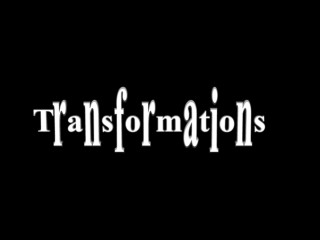DownloadDownload PresentationReflection

# Reflection

Download Presentation## Reflection

- - - - - - - - - - - - - - - - - - - - - - - - - - - E N D - - - - - - - - - - - - - - - - - - - - - - - - - - -
##### Presentation Transcript

1. Reflection Reflection

2. To reflect harder shapes, we reflect each of their corners separately and then join the reflected points I O Reflection produces congruent shapes

3. To reflect harder shapes, we reflect each of their corners separately and then join the reflected points O I

4. Rotation

5. What is the meaning of Rotation? • Rotate the rectangle: • 90° • Clockwise • About C O c Centre of Rotation I Rotation is a Transformation

6. What is the meaning of Rotation? • Rotate the triangle: • 90° • Anti-clockwise • About C O I c Rotation produces congruent shapes

7. Formal Rotation

8. How do we rotate a shape in general? • Rotate this shape: • 60° • Anti-clockwise • About C O I 60° c

9. How do we rotate a shape in general? • Rotate this shape: • 60° • Anti-clockwise • About C O I 60° c

10. Sliding Translation = Horizontal Steps vector Vertical Steps I O

11. 01234567891011121314151617181920212223 Enlarge this rectangle by a scale factor of 2 about the marked centre of enlargement C Centre of Enlargement

12. 01234567891011121314151617181920212223 Enlarge this rectangle by a scale factor of 2 about the marked centre of enlargement Can you see where the rest of the shape will be? C

13. 01234567891011121314151617181920212223 Enlarge this rectangle by a scale factor of 2 about the marked centre of enlargement Can you see where the rest of the shape will be? C

14. 01234567891011121314151617181920212223 Enlarge this rectangle by a scale factor of 2 about the marked centre of enlargement Can you see where the rest of the shape will be? I 0 C

15. 01234567891011121314151617181920212223 Enlarge this shape by a scale factor of 3 about the marked centre of enlargement Can you see where the rest of the shape will be? C

16. 01234567891011121314151617181920212223 Enlarge this shape by a scale factor of 3 about the marked centre of enlargement C

17. 01234567891011121314151617181920212223 Enlarge this shape by a scale factor of 3 about the marked centre of enlargement C

18. 01234567891011121314151617181920212223 Enlarge this shape by a scale factor of 3 about the marked centre of enlargement C

19. Enlarge this shape by a scale factor of 3 about the marked centre of enlargement I C O

20. The Different Positions of the Centre of Enlargement

21. Finding The Centre of Enlargement

22. Scale Factor Pairs

23. x 2 What is the scale factor from A to B? x ½ What is the scale factor from B to A? B C A

24. x 1 3 What is the scale factor from A to B? x 3 What is the scale factor from B to A? B A C

25. x x 2 3 3 2 What is the scale factor from A to B? What is the scale factor from B to A? C A B The scale factors which transform object to image and vice versa are always reciprocals of each other

26. Negative Scale Factors

27. What is the meaning of a negative scale factor?

28. Enlarge object A by a scale factor of -1 +ve -ve C A B What is the scale factor from B to A? What other single transformation would have produced the same result from A to B?

29. Enlarge object A by a scale factor of -1 C A B The Enlargement with scale factor -1 and a given centre of enlargement C is the same as a rotation by 180° about C , and C is also known as centre of symmetry

30. 1 2 Enlarge object A by a scale factor of -1 -2 C A B What is the scale factor from B to A? What combination of transformations would have produced the same result from A to B?

31. Summary on Transformations

32. REFLECTION • Object • Line of reflection • Congruent Image • Orientation is not maintained ROTATION • Object • Centre of Rotation • Direction of Rotation • Amount of Rotation • Congruent Image • Orientation is not maintained TRANSLATION • Object • Vector • Congruent Image • Orientation is maintained ENLARGEMENT • Object • Scale Factor • Centre of Enlargement • Similar Image • Orientation is maintained or • turned “upside down”